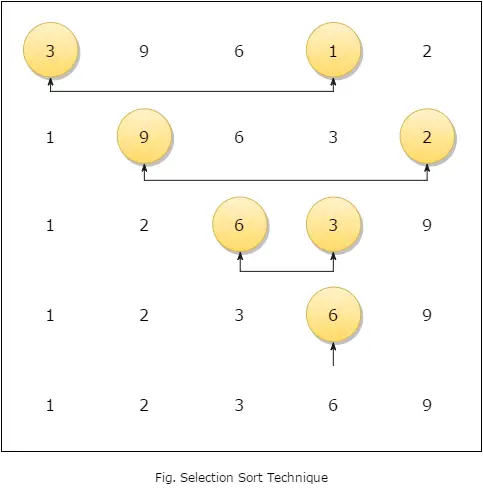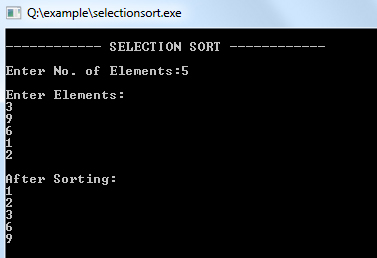# Selection Sort Algorithm

The selection is a straightforward process of sorting values. In this method, to sort the data in ascending order, the 0th element is compared with all other elements. If the 0th element is found to be greater than the compared element, the two values get interchanged. In this way after the first iteration, the smallest element is placed at 0th position. The technique is repeated until the full array gets sorted.

The below-given figure shows how Selection Sort works:Example:

``````#include <iostream>
using namespace std;

int main()
{
int a, i, n, p, k, min, loc, tmp;

cout << "\n------------ SELECTION SORT ------------ \n\n";
cout << "Enter No. of Elements:"; cin >> n;

cout << "\nEnter Elements:\n";
for (i = 1; i <= n; i++) { cin >> a[i];
}

for (p = 1; p <= n - 1; p++) // Loop for Pass
{
min = a[p]; // Element Selection
loc = p;

for (k = p + 1; k <= n; k++) // Finding Min Value { if (min > a[k]) {
min = a[k];
loc = k;
}
}

tmp = a[p];
a[p] = a[loc];
a[loc] = tmp;
}
cout << "\nAfter Sorting: \n";
for (i = 1; i <= n; i++) {
cout << a[i] << endl;
}
}``````

Output: